## The meter was originally defined so that the period of a meter-long simple pendulum would be exactly 2.00 second. (Was your measured value c

Question

The meter was originally defined so that the period of a meter-long simple pendulum would be exactly 2.00 second. (Was your measured value close to this?) Given the relationship, T^2 alpha L (T^2 is proportional to L) what would be the length of a simple pendulum, in centimeters, with a period of exactly one second?

in progress 0
2 months 2021-07-30T09:47:38+00:00 1 Answers 1 views 0

1. Explanation:

Given that,

Initial length of simple pendulum,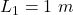Initial time period,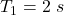We need to find the length of the simple pendulum when the period is exactly 1 second.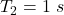We know that the time period of simple pendulum is given by :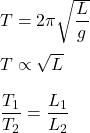Put all values and find L₂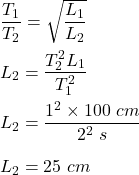So, the length of the pendulum with a period of exactly one second is 25 cm.